Geometry Help: Proposed Problem 40. Level: High School, SAT Prep, College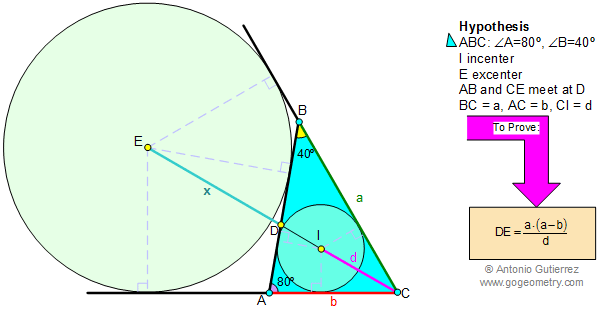SUGGESTIONS for Problem 40 DEFINITION 1. Angle is the figure formed by two rays with a common end point. Congruent angles are angles that have the exact same measure (the same number of degrees). Angle Bisector is a ray that divides the angle into two congruent adjacent angles.DEFINITION 2. Triangle is a three side polygon. Polygon is a closed plane figure with n sides. Altitude is the perpendicular line segment from one vertex to the line that contains the opposite side.PROPOSITION 1. The sum of the measures of the three angles of a triangle is 180.PROPOSITION 2. The measure of an exterior angle of a triangle equals the sum of the measures of the two non-adjacent interior angles.PROPOSITION 3. The sum of the measures of the acute angles of a right triangle is 90 (complementary).PROPOSITION 4. Triangle Congruence A.S.A. If two angles and the included side of one triangle are congruent to the corresponding parts of another, then the triangles are congruent.PROPOSITION 5. Any point on the bisector of an angle is equidistant from the sides of the angle. Also conversePROPOSITION 6. Triangle Congruence S.A.S. If two sides and the included angle of one triangle are congruent to the corresponding parts of another, then the triangles are congruent.PROPOSITION 7. Isosceles triangle: If two sides of a triangle are congruent, the angles opposite these sides are congruent. Also converse.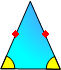PROPOSITION 8. An equilateral triangle is equiangular: 60-60-60. Also converse.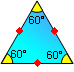DEFINITION 3. Circle is the set of all points in a plane that are at the same distance from a fixed point called the center. Tangent of a circle is a line that touches the circle at one and only one point no matter how far produced. PROPOSITION 9. If a line is tangent to a circle, it is perpendicular to a radius at the point of tangency.PROPOSITION 10. The bisectors AD, BF and CE of the angles of a triangle ABC meet in a point I, which is equidistant from the sides of the triangle. The incircle is the inscribed circle of a triangle. The center of the incircle is called the incenter, and the radius of the circle is called the inradius.PROPOSITION 11. An excircle of the triangle is a circle lying outside the triangle, tangent to one of its sides and tangent to the extensions of the other two. The center of an excircle is the intersection of the internal bisector of one angle and the external bisectors of the other two. The center of an excircle is called the excenter, and the radius of the circle is called the exradius.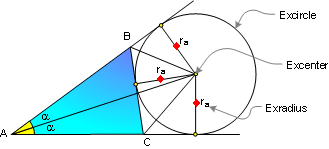PROPOSITION 12. A central angle is measured by its intercepted arc.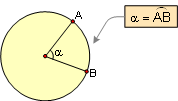PROPOSITION 13. An inscribed angle is measured by one-half its intercepted arc.DEFINITION 4. A cyclic quadrilateral is a quadrilateral whose vertices all lie on a single circle. PROPOSITION 14.1 Opposite angles of a cyclic (inscribed) quadrilateral are supplementary. Also converse.PROPOSITION 14.2 A quadrilateral is cyclic if one side subtends congruent angles at the two opposite vertices. Also converse.DEFINITION 5. Similar Triangles are triangles whose corresponding angles are congruent and whose corresponding sides are in proportion.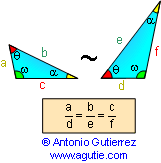PROPOSITION 15. Triangle Similarity AA. If two angles of one triangle are congruent to two angles of another triangle, the two triangles are similar.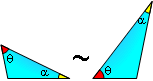Home | Geometry | Search | Problems | Open Problems | 10 Problems | Email | By Antonio Gutierrez